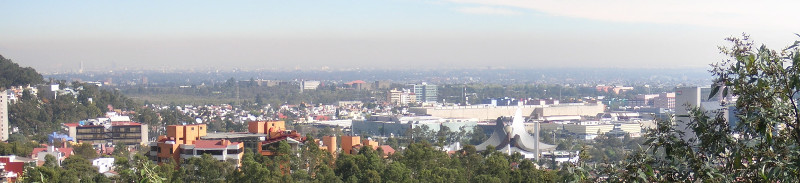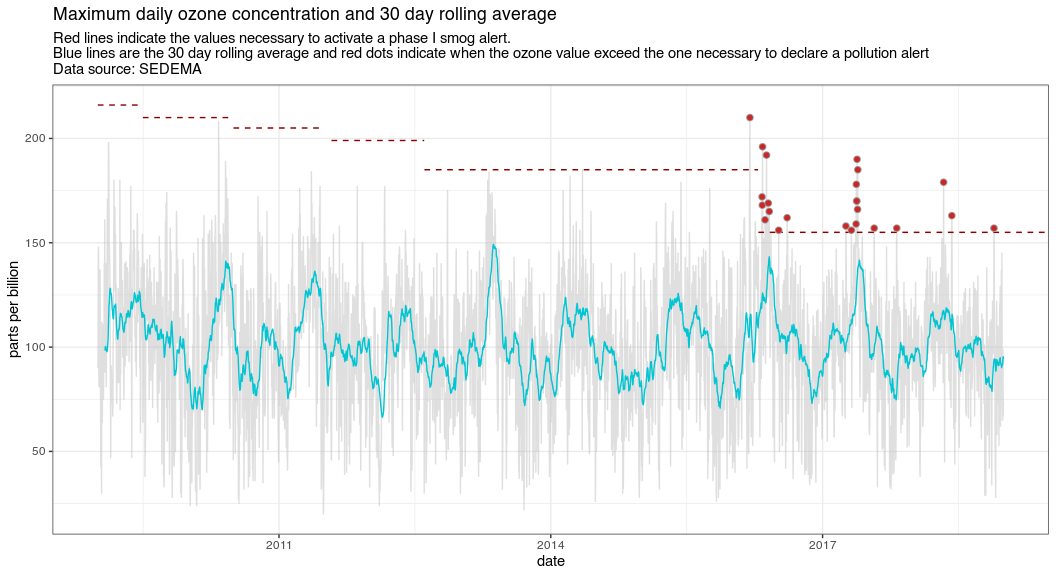aire.zmv is an R package for downloading air quality data from the Mexico City metro area. This package can download real-time, daily maximum, minimum, or hourly average data for each of the pollution measuring stations or geographical zones in the Zona Metropolitana del Valle de México (greater Mexico City). It also includes the locations of all the measuring stations and zones, and a function to perform inverse distance weighting modified to work with wind direction.

## Installation

You can always install the development version from GitHub:

if (!require(devtools)) {
install.packages("devtools")
}
devtools::install_github('diegovalle/aire.zmvm')

To install the most recent package version from CRAN type:

install.packages("aire.zmvm")
library(aire.zmvm)

Note that the version on CRAN might not reflect the most recent changes made to this package.

## Usage

Create a time series of ozone levels in ppb from 2009 to 2018 and highlight the phase I smog alerts.

## Auto-install required R packages
if (!require("pacman")) install.packages("pacman")

o3 <- get_station_data(criterion = "MAXIMOS", # Can be one of MAXIMOS (daily maximum),
# MINIMOS (daily minimum),
# or HORARIOS (hourly average)
pollutant = "O3", # Can be one of "SO2", "CO", "NOX", "NO2", "NO", "O3",
# "PM10", "PM25", "WSP", "WDR", "TMP", "RH"
year = 2009:2018) # A numeric vector, the earliest year allowed is 1986
# Daily max among all base stations
o3_max <- o3 %>%
group_by(date) %>%
summarise(max = ifelse(all(is.na(value)),
NA,
base::max(value, na.rm = TRUE))) %>%
na.omit()

# ozone threshold for declaring a 'smog alert' and the dates during which they
# were valid
contingencia_levels <- data.frame(ppb = c(216, 210, 205,
199, 185, 155, 155),
start = c(2009, 2009.4973, 2010.4973, 2011.5795,
2012.6052,  2016.291, 2016.4986),
end = c(2009.4973, 2010.4945, 2011.4945,
2012.6025,    2016.2883, 2016.4959, Inf))
max_daily_df <- tsdf(ts(o3_max$max, start = c(2009,1), frequency = 365.25)) contingencia <- o3_max contingencia$date <- max_daily_df$x contingencia$contingencia <- case_when(
contingencia$date > 2012.6052 & contingencia$max > 185 ~ TRUE,
contingencia$date > 2016.291 & contingencia$max > 155 ~ TRUE,
TRUE ~ FALSE
)

knitr::kable(head(o3))
date station_code pollutant unit value
2009-01-01 ACO O3 ppb 67
2009-01-02 ACO O3 ppb 71
2009-01-03 ACO O3 ppb 112
2009-01-04 ACO O3 ppb 91
2009-01-05 ACO O3 ppb 70
2009-01-06 ACO O3 ppb 71
ggplot(max_daily_df,
aes(x = x, y = y)) +
geom_line(colour = "grey75", alpha = .5) +
stat_rollapplyr(width = 30, align = "right", color = "#01C5D2") +
geom_segment(data = contingencia_levels,
aes(x=start, y=ppb, xend=end, yend=ppb), color="darkred",
linetype = 2)  +
geom_point(data=filter(contingencia, contingencia == TRUE),
aes(x=date, y=max), color = "#999999",
size = 2, shape = 21, fill = "firebrick3" ) +
xlab("date") +
ylab("parts per billion") +
scale_x_continuous(breaks = c(2011, 2014, 2017)) +
ggtitle("Maximum daily ozone concentration and 30 day rolling average",
subtitle = paste0("Red lines indicate the values necessary to ",
"activate a phase I smog alert. \nBlue lines are ",
"the 30 day rolling average and red dots indicate ",
"when the ozone value exceed the one necessary to ",
"declare a pollution alert\nData source: SEDEMA")) +
theme_bw()